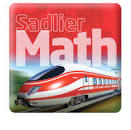# MATH

•Chapter 6

Why is place value important?

*In this chapter, we will be learning about place value to 100.

Words we will learn to know:

tens
ones
ten-frame
ten rod
tens number
two-digit number
hundred chart
count by 2's
count by 5's
count by 10's

*Go on Freckle Math as often as possible to practice those facts!!
Click on Freckle below to access Freckle Math: